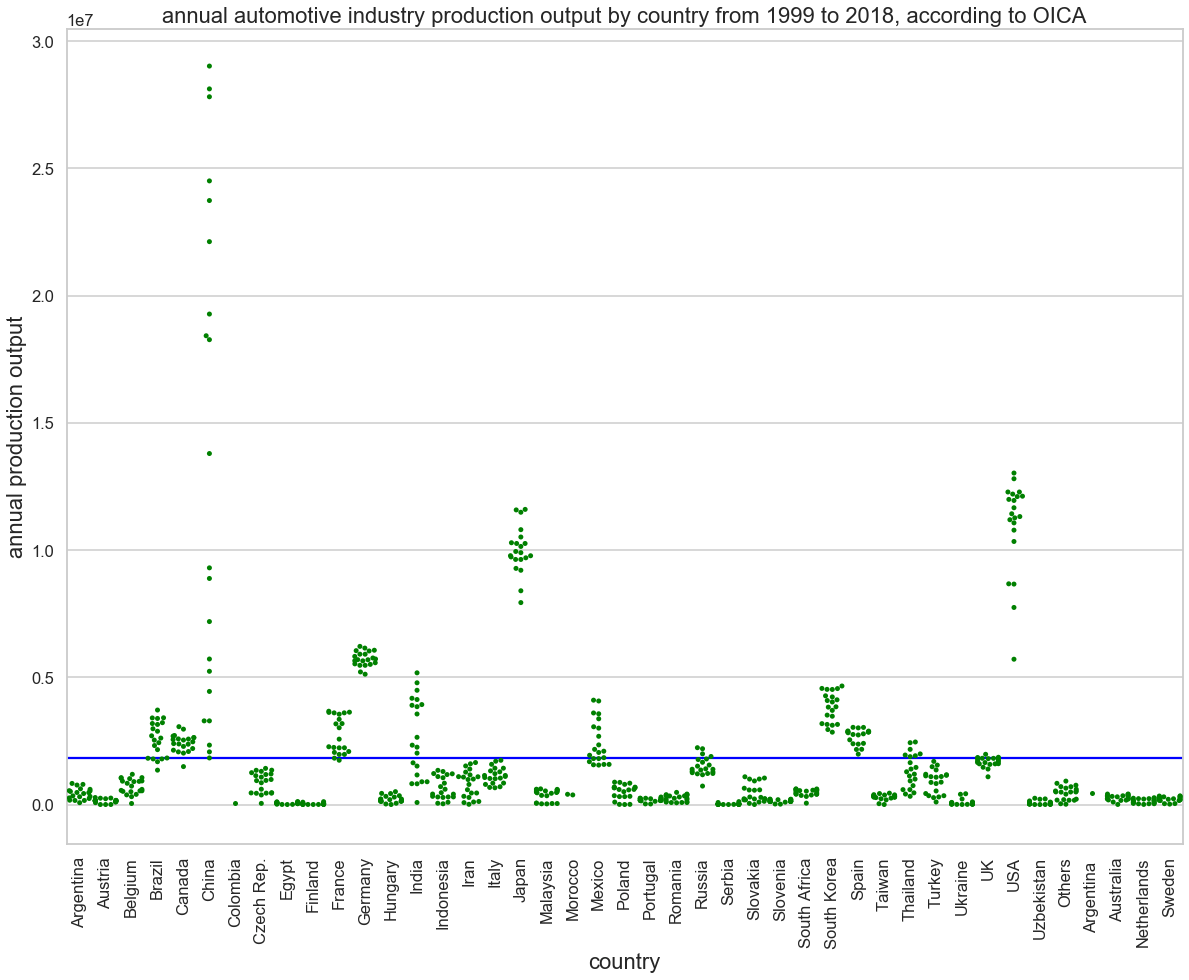# Python 中的 Seaborn 简介``````import pandas
import seaborn
import numpy
import matplotlib.pyplot as plt``````
``````# 让我们也看看尾部
data_df.tail()``````
``````# 让我们试试 .describe()
data_df.describe()``````
``````plt.figure(figsize=(20,15))
plot1 = seaborn.scatterplot(x="year",y="output ",hue="country ", data=data_df)
plot1.set_title("production output by year (OICA data)", fontsize=22)
plot1.set_xlabel("year",fontsize=16)``````
`文本(0, 0.5, '输出')`
``````plt.figure(figsize=(20,15))
plt.xticks(rotation=90)
plot2 = seaborn.boxplot(x="country ",y="output ",data=data_df)
plot2.set_title("annual production output distribution by nation, 1999 - 2018 (OICA data)",fontsize=22)
plot2.set_xlabel("country",fontsize=16)
plot2.set_ylabel("annual production output",fontsize=16)``````
`Text(0, 0.5, '年产量')`
``````plt.figure(figsize=(20,15))
plt.xticks(rotation=90)

seaborn.set_style("whitegrid")
seaborn.set_context("talk")

plot3 = seaborn.swarmplot(x="country ",y="output ",data=data_df,color="green")

plot3.set_title("annual automotive industry production output by country from 1999 to 2018, according to OICA",fontsize=22)

plot3.set_xlabel("country",fontsize=22)

plt.axhline(data_df["output "].mean(),color="blue")``````
`<matplotlib.lines.Line2D 位于 0x2b02ec39b88>`
``````plt.figure(figsize=(20,15))

seaborn.set_context("paper")

plot4 = seaborn.lineplot(x="year",y="output ",hue="country ",data=data_df)

plot4.set_title("OICA automotive industry production output time series, 1999 - 2018",fontsize=22)

plot4.set_xlabel("year",fontsize=22)
plot4.set_ylabel("production output [units]",fontsize=22)``````
`Text(0, 0.5, '产量[单位]')`